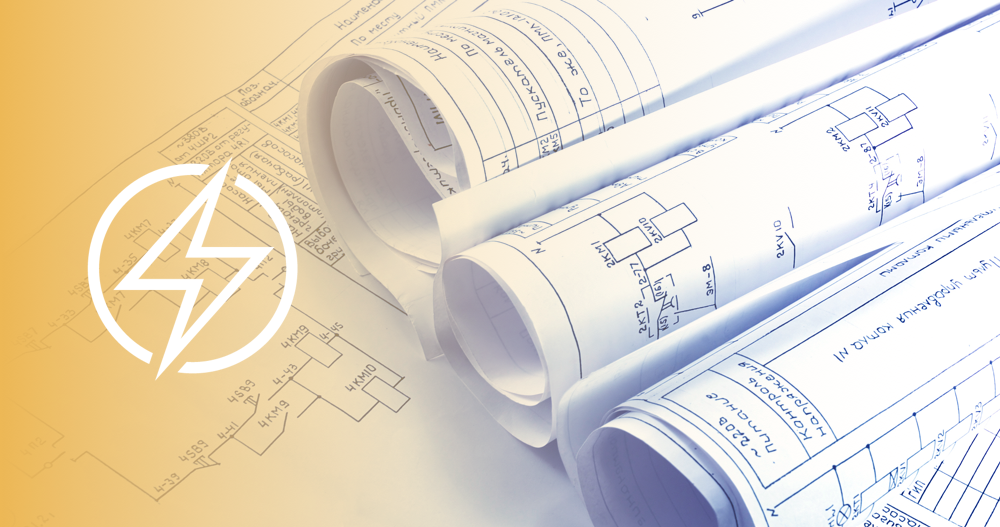Take the First Step Toward Your Electrical Career Now!

# ​13 Common Electrical Terms Every Electrician Should KnowIf you are thinking about becoming an electrician, there are a few terms you will be required to know. You can get a head start on your electrical training by reviewing some of the most important ones here.

• Amp (Ampere): The unit of intensity of electrical current, otherwise known as the measure of electrical flow.
• Capacitor: The capacitor is an electronic component made of two close conductors that store electric charge. The plates accumulate electric charge when connected to a power source, one accumulating positive charge and another negative charge. Capacitance is the amount of electric charge stored in the capacitor at 1 Volt. The capacitor disconnects current in direct current (DC) circuits and short circuit in alternating current (AC) circuits.
• Electrical current: The flow rate of electrical charge in an electric field, usually in an electrical circuit.
• Electric power: The rate of energy consumption in an electrical circuit. Electric power is measured in units of watts.
• Electrical resistance: An electrical quantity that measures how the device or material reduces the electric current flowing through it. The resistance is measured in ohms.
• Ohm: The unit of electrical resistance and impedance abbreviated with the omega symbol. Resistance is the opposition a substance offers to the passage of electrical current.
• Service: The conductors and equipment for delivering electrical energy from the supply system to the wiring system of the premises served.
• Volt: The unit of electromotive force that measures electrical pressure. Voltage is the effective difference of potential between any two conductors of the circuit concerned. The Nominal Voltage is the value assigned to a circuit to designate its voltage class, although the actual voltage of the circuit can vary.
• Watt: The unit of power or rate of work represented by a current of one ampere under a pressure of one volt. Wattage ratings of lamps measure the power consumption, not the illuminating capability.
• Electric charge: Electric charge generates the electric field. The electric charge influences other electric charges with electric force and is also influenced by other charges with the same force in the opposite direction. There are two types of electrical charges:
• Positive charge: A positive charge has more protons than electrons. Positive charges attract negative charges and are attracted by other negative charges. They repel and are repelled by other positive charges.
• Negative charge: A negative charge has more electrons than protons. Negative charges attract positive charges and are attracted by other positive charges. They repel and are repelled by other negative charges.
• Power efficiency: Power efficiency is the ratio of output power divided by the input power.
• Power factor: In AC circuits, the power factor is the ratio of real power used to do work and the apparent power supplied to the circuit. The power factor is given values that range from 0 to 1.
• The power factor is 0 when all of the power is reactive power with no real power (usually an inductive load).
• The power factor is 1 when all of the power is real power with no reactive power (resistive load).
• Resistor: Resistor is an electrical component that reduces the electrical current. The resistor’s ability to reduce the current is called resistance and is measured in units of ohms.

Find a local electrical trade school now.

Walk into the classroom prepared by knowing these terms. You’ll be one step ahead in your electrical training and one step closer in your career as an electrician.

Want more? Try our Electrical NEC & Electrical Theory Practice Tests